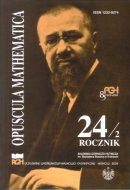Opuscula Math. 24, no. 2 (2004), 177-180

Opuscula Mathematica

# Nearly perfect sets in products of graphs

Maria Kwaśnik
Monika Perl

Abstract. The study of nearly perfect sets in graphs was initiated in [Dunbar J. E., Harris F. C., Hedetniemi S. M., Hedetniemi S. T., McRae A. A., Laskar R. C.: Nearly perfect sets in graphs. Discrete Mathematics 138 (1995), 229-246]. Let $$S\subseteq V(G)$$. We say that $$S$$ is a nearly perfect set (or is nearly perfect) in $$G$$ if every vertex in $$V(G)-S$$ is adjacent to at most one vertex in $$S$$. A nearly perfect set $$S$$ in $$G$$ is called maximal if for every vertex $$u\in V(G)-S$$, $$S\cup \{u\}$$ is not nearly perfect in $$G$$. The minimum cardinality of a maximal nearly perfect set is denoted by $$n_p(G)$$. It is our purpose in this paper to determine maximal nearly perfect sets in two well-known products of two graphs; i.e., in the Cartesian product and in the strong product. Lastly, we give upper bounds of $$n_p(G_1\times G_2)$$ and $$n_p(G_1\otimes G_2)$$, for some special graphs $$G_1$$, $$G_2$$.

Keywords: dominating sets, product of graphs.

Mathematics Subject Classification: 05C69, 05C70.

Full text (pdf)

• Maria Kwaśnik
• Technical University of Szczecin, Institute of Mathematics, al. Piastów 48/49, 70-310 Szczecin, Poland
• Monika Perl
• Technical University of Szczecin, Institute of Mathematics, al. Piastów 48/49, 70-310 Szczecin, Poland